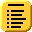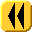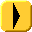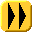## Chapter 14: Initial-Value ProblemsIf a first-order ordinary differential equation in y(t) is prescribed to have a fixed value y(t0) = y0, then problem of finding a function y(t) which satisfies both of these conditions is termed an initial-value problem (IVP).

To begin, we will look specifically at solving linear first-order ODEs of the form:

y(1)(t) = a(t) y(t) + b(t)

where a(t) and b(t) are known functions.

This topic looks at a three techniques for solving first-order linear IVPs, each of which is an improvement on the previous. Then we will look at other improvements and a technique for converting a higher-order IVP into a system of first-order IVPs is given.